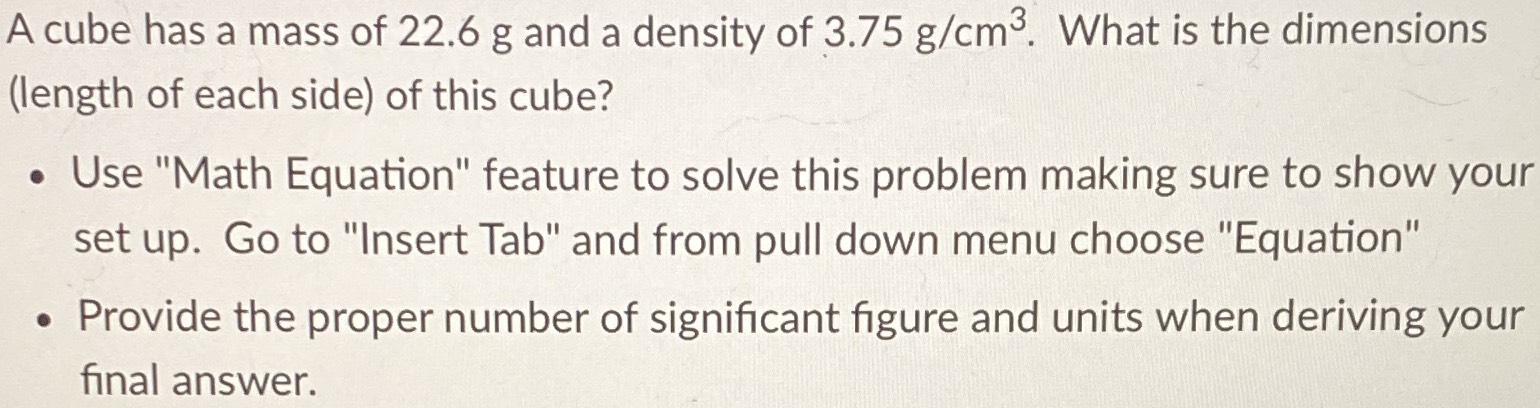### ¿Todavía tienes preguntas de matemáticas?

Pregunte a nuestros tutores expertos
Algebra
PreguntaA cube has a mass of $$22.6 g$$ and a density of $$3.75 g / cm ^ { 3 }$$ . What is the dimensions (length of each side) of this cube? - Use "Math Equation" feature to solve this problem making sure to show your set up. Go to "Insert Tab" and from pull down menu choose "Equation" - Provide the proper number of significant figure and units when deriving your final answer.

$$x ^ { 3 } \cdot 3.75 = 22.6 \quad : \quad x = \sqrt[ 3 ] { \frac { 452 } { 75 } }$$
$$\sqrt[ 3 ] { \frac { 452 } { 75 } } = 1.81980 \ldots$$